# Array Indexing

+

Array Indexing adds the capability to create global indexes on array elements and optimizes the execution of queries involving array elements.

This is a huge leap from the previous versions where secondary indexes could only be created and subsequently queried on whole arrays. You can now create an index of array elements ranging from plain scalar values to complex arrays or JSON objects nested deeper in the array.

## Syntax

`create-index ::= CREATE INDEX index-name ON keyspace-ref '(' index-key [ index-order ] [ ',' index-key [ index-order ] ]* ')' [ where-clause ] [ index-using ] [ index-with ]`index-name

Specify a unique name to identify the index.

### Keyspace Reference

`keyspace-ref ::= keyspace-path | keyspace-partial`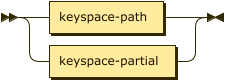`keyspace-path ::= [ namespace ':' ] bucket [ '.' scope '.' collection ]`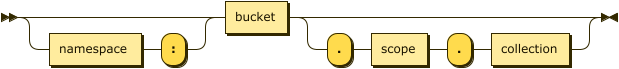`keyspace-partial ::= collection`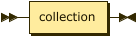The simple name or fully-qualified name of the keyspace on which to create the index. Refer to the CREATE INDEX statement for details of the syntax.

### Index Key

`index-key ::= expr | array-expr`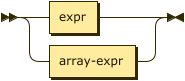Refers to an attribute name or a scalar function or an ARRAY expression on the attribute. This constitutes an index-key for the index.

expr

A N1QL expression over any fields in the document. This cannot use constant expressions, aggregate functions, or sub-queries.

### Array Expression

`array_expr ::= full-array-expr | simple-array-expr`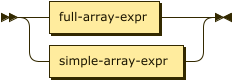The query predicate which appears in the WHERE clause of a SELECT, UPDATE, or DELETE statement must have exactly the same format as the variable in the array index key. See Format of Query Predicate for details.
 Currently, array indexing is limited to using only one index-key with the array expression. To create an array index involving multiple array elements or multiple arrays, use a full array expression whose variable expression is constructed as a compound object constituted with different elements of the same array or multiple arrays. Subsequent SELECT or DML statements must use similar compound objects in the WHERE clause to use the array index. See Examples below. For an UNNEST scan to use an index, the leading key of the index definition must be an appropriate ARRAY index key. In Couchbase Server 6.0.1 and later, the UNNEST scan can generate index spans on other non-leading index keys when appropriate predicates exist.

#### Full Array Expression

`full-array-expr ::= ( ALL | DISTINCT ) ARRAY var-expr FOR var ( IN | WITHIN ) expr [ ',' var ( IN | WITHIN ) expr ]* [ WHEN cond ] END`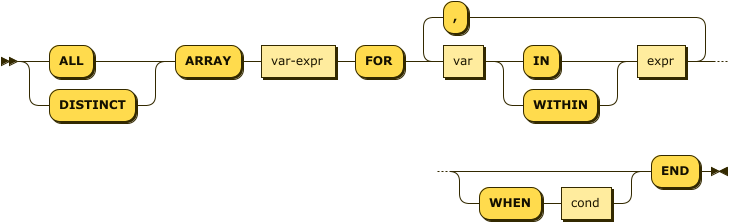The `ARRAY` operator lets you map and filter the elements or attributes of a collection, object, or objects. It evaluates to an array of the operand expression that satisfies the WHEN clause, if specified.

var-expr

A function of the `var` variable used in the FOR clause.

var

Represents elements in the array specified by `expr`.

expr

Evaluates to an array of objects, elements of which are represented by the `var` variable.

cond

Specifies predicates to qualify the subset of documents to include in the index array.

 The `var-expr` itself can be a nested array expression. This enables creating array indexes on nested array fields. See Examples below.

#### Simple Array Expression

`simple-array-expr ::= ( ALL | DISTINCT ) expr`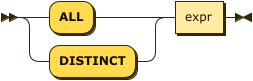Couchbase Server 5.0 and later provides a simpler syntax for array indexing when all array elements are indexed as is, without needing to use the `ARRAY` operator in the index definition.

expr

An array field name, or an expression that can evaluate to an array. In this case, all elements of the array are indexed.

### Index Order

`index-order ::= ASC | DESC`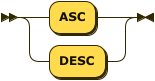Specifies the sort order of the index key.

`ASC`

The index key is sorted in ascending order.

`DESC`

The index key is sorted in descending order.

This clause is optional; if omitted, the default is `ASC`.

### WHERE Clause

`where-clause ::= WHERE cond`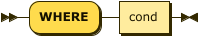cond

Specifies WHERE clause predicates to qualify the subset of documents to include in the index.

### USING Clause

`index-using ::= USING GSI`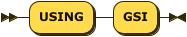The index type for an array index must be Global Secondary Index (GSI). The `USING GSI` keywords are optional and may be omitted.

### WITH Clause

`index-with ::= WITH expr`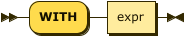Use the WITH clause to specify additional options.

expr

An object specifying additional options for the query.

See the CREATE INDEX statement for more details on the syntax.

## Format of Query Predicate

The query predicate which appears in the WHERE clause of a SELECT, UPDATE, or DELETE statement must have exactly the same format as the variable in the array index key.

A SELECT query or DML statement that needs to use the array index can use different variable names in the query from those used in the array index definition.

Consider the following expressions used in a CREATE INDEX statement:

``````DISTINCT ARRAY f(x) FOR x IN expr1 END; (1)

DISTINCT ARRAY f(x) FOR x WITHIN expr1 END; (2)``````

And the following expressions used in the SELECT statement WHERE clause:

``````ANY x IN expr2 SATISFIES g(x) END; (3)

ANY x WITHIN expr2 SATISFIES g(x) END (4)``````

The following dependencies must be satisfied for the Query service to consider the array index:

• The index keys used in CREATE INDEX must be used in the WHERE clause.

• `expr2` in ➂ and ➃ must be equivalent to `expr1` in ➀ and ➁. This is a formal notion of equivalence. For example, if they are the same expressions, or equivalent arithmetic expressions such as `(x+y)` and `(y+x)`.

• `g(x)` in ➂ and ➃ must be sargable for `f(x)` in ➀ and ➁. In other words, if there were a scalar index with key `f(x)`, then that index would be applicable to the predicate `g(x)`. For example, the index key `UPPER(x)` is sargable for the predicate `UPPER(x) LIKE "John%"`.

• IN vs. WITHIN: Index key ➀ can be used for query predicate ➂. Index key ➁ can be used for both query predicates ➂ and ➃.

 Index key ➁ is strictly more expensive than index key ➀, for both index maintenance and query processing. Index key ➁ and query predicate ➃ are very powerful. They can efficiently index and query recursive trees of arbitrary depth.

## Examples

The following examples use the travel-sample bucket that is shipped with Couchbase Server.

Example 1. Indexing all DISTINCT elements in an array
Index: Create an index on all schedules
``````CREATE INDEX idx_sched
ON `travel-sample`.inventory.route
( DISTINCT ARRAY v.flight FOR v IN schedule END );``````
Query: Find the list of scheduled 'UA' flights
``````SELECT * FROM `travel-sample`.inventory.route
WHERE ANY v IN schedule SATISFIES v.flight LIKE 'UA%' END;``````
Example 2. Partial index (with WHERE clause) of individual attributes from selected elements (using WHEN clause) of an array
Index: Create an index on flights from San Francisco scheduled in the first 4 days of the week
``````CREATE INDEX idx_flight_sfo
ON `travel-sample`.inventory.route
( ALL ARRAY v.flight FOR v IN schedule WHEN v.day < 4 END )
WHERE sourceairport = "SFO";``````
Query: Find the list of scheduled 'UA' flights on day 1
``````SELECT * FROM `travel-sample`.inventory.route
WHERE sourceairport = "SFO" (1)
AND ANY v IN schedule SATISFIES (v.flight LIKE 'UA%') (2)
AND (v.day=1) END; (3)``````

In this example, the Index qualifies for the Query because:

 1 The Query predicate `sourceairport = "SFO"` matches that of the partial index WHERE clause. 2 The ANY operator uses the index key `v.flight` on which the Index is defined. 3 The ANY-SATISFIES condition `v.day=1` in the Query is sargable to that in the index definition WHEN clause `v.day < 4`.
Example 3. Compound array index with individual elements of an array and other non-array fields
Index: Create an index on scheduled flight IDs and number of stops
``````CREATE INDEX idx_flight_stops
ON `travel-sample`.inventory.route
( stops, DISTINCT ARRAY v.flight FOR v IN schedule END );``````
Query: Find the list of scheduled 'FL' flights that have one or more stops
``````SELECT * FROM `travel-sample`.inventory.route
WHERE stops >=1
AND ANY v IN schedule SATISFIES v.flight LIKE 'FL%' END;``````
Example 4. Indexing the individual elements of nest arrays

Use the DISTINCT ARRAY clause in a nested fashion to index specific attributes of a document when the array contains other arrays or documents that contain arrays. For example,

Update: Create a nested array `special_flights`
``````UPDATE `travel-sample`.inventory.route
SET schedule = {"day" : 7, "special_flights" :
[ {"flight" : "AI444", "utc" : "4:44:44"},
{"flight" : "AI333", "utc" : "3:33:33"}
] }
WHERE destinationairport = "CDG" AND sourceairport = "TLV";``````
Index: Create a partial index on a nested array `special_flights`
``````CREATE INDEX idx_nested ON `travel-sample`.inventory.route
(DISTINCT ARRAY
(DISTINCT ARRAY y.flight (1)
FOR y IN x.special_flights END)
FOR x IN schedule END);``````
 1 In this case, the inner ARRAY construct is used as the `var_expr` for the outer ARRAY construct in the N1QL Syntax above.
Query A: Use nested ANY operator to use the index
``````SELECT count(*) FROM `travel-sample`.inventory.route
WHERE ANY x in schedule SATISFIES
(ANY y in x.special_flights SATISFIES y.flight IS NOT NULL END)
END;``````

This query returns 3 results, as there are 3 routes with special flights.

Query B: Use UNNEST operators to use the index
``````SELECT count(*) FROM `travel-sample`.inventory.route
UNNEST schedule AS x
UNNEST x.special_flights AS y
WHERE y.flight IS NOT NULL;``````

This query returns 6 results, as there are 3 routes with 2 special flights each.

Example 5. Array Index with multiple elements of an array
Index: Create an index on `flight` and `day` fields in `schedule`
``````CREATE INDEX idx_flight_day ON `travel-sample`.inventory.route
(DISTINCT ARRAY [v.flight, v.day] FOR v IN schedule END);``````
Query: Find the list of scheduled 'US681' flights on day 2
``````SELECT meta().id FROM `travel-sample`.inventory.route
WHERE ANY v in schedule SATISFIES [v.flight, v.day] = ["US681", 2] END;``````
Example 6. Indexing all elements in an array using simplified syntax
Index: Create an index on all schedules using simplified array index syntax
``````CREATE INDEX idx_sched_simple
ON `travel-sample`.inventory.route (ALL schedule);``````
Query A: Find details of all route documents matching a specific schedule
``````SELECT * FROM `travel-sample`.inventory.route
WHERE ANY v IN schedule
SATISFIES v = {"day":2, "flight": "US681", "utc": "19:20:00"} END; (1)``````
 1 Elements of the schedule array are objects, and hence the right side value of the predicate condition should be a similarly structured object.
Query B: Find details of all route documents matching a specific schedule
``````SELECT * FROM `travel-sample`.inventory.route t
UNNEST schedule sch
WHERE sch = {"day":2, "flight": "US681", "utc": "19:20:00"};``````

This is a variant of Query A using UNNEST in the SELECT statement.

## Covering Array Index

Covering indexes are an efficient method of using an Index for a particular query, whereby the index itself can completely cover the query in terms of providing all data required for the query. Basically, it avoids the fetch phase of the query processing and related overhead in fetching the required documents from data-service nodes. For more details, see Covering Indexes.

Array indexing requires special attention to create covering array indexes. In general, the array field itself should be included as one of the index keys in the CREATE INDEX definition. For instance, in Example 1, the Index does not cover the Query because the Query projection list includes * which needs to fetch the document from the Data Service.

Example 7. Covering Array Index
Index I: Creating a Covering Array Index
``````CREATE INDEX idx_sched_cover ON `travel-sample`.inventory.route
(DISTINCT ARRAY v.flight FOR v IN schedule END, schedule);``````

The index keys of an index must be used in the WHERE clause of a DML statement to use the index for that query. In the SELECT or DML WHERE clause, Covering Array Indexes can be used by the following operators:

Query A: Covering Array Index using the ANY clause
``````EXPLAIN SELECT meta().id FROM `travel-sample`.inventory.route
USE INDEX (idx_sched_cover) (1)
WHERE ANY v IN schedule SATISFIES v.flight LIKE 'UA%' END;``````
 1 In this example, Query A needs Index I to cover it because the query predicate refers to the array `schedule` in the ANY operator.
Result
``````[
{
"plan": {
"#operator": "Sequence",
"~children": [
{
"#operator": "DistinctScan",
"scan": {
"#operator": "IndexScan3",
"bucket": "travel-sample",
"covers": [
"cover ((distinct (array (`v`.`flight`) for `v` in (`route`.`schedule`) end)))",
"cover ((`route`.`schedule`))",
"cover ((meta(`route`).`id`))"
],
"filter": "cover (any `v` in (`route`.`schedule`) satisfies ((`v`.`flight`) like \"UA%\") end)",
"filter_covers": {
"cover (any `v` in (`route`.`schedule`) satisfies ((\"UA\" <= (`v`.`flight`)) and ((`v`.`flight`) < \"UB\")) end)": true,
"cover (any `v` in (`route`.`schedule`) satisfies ((`v`.`flight`) like \"UA%\") end)": true
},
"index": "idx_sched_cover",
...
}
}
]
}
}
]``````
Query B: Covering Array Index using the ANY AND EVERY clause
``````EXPLAIN SELECT meta().id FROM `travel-sample`.inventory.route
USE INDEX (idx_sched_cover)
WHERE ANY AND EVERY v IN schedule SATISFIES v.flight LIKE 'UA%' END;``````
Result
``````[
{
"plan": {
"#operator": "Sequence",
"~children": [
{
"#operator": "DistinctScan",
"scan": {
"#operator": "IndexScan3",
"bucket": "travel-sample",
"covers": [
"cover ((distinct (array (`v`.`flight`) for `v` in (`route`.`schedule`) end)))",
"cover ((`route`.`schedule`))",
"cover ((meta(`route`).`id`))"
],
"filter": "any and every `v` in cover ((`route`.`schedule`)) satisfies ((`v`.`flight`) like \"UA%\") end",
"index": "idx_sched_cover",
...
}
}
]
}
}
]``````
Query C: Covering Array Index using the UNNEST clause and aliasing
``````EXPLAIN SELECT meta(t).id FROM `travel-sample`.inventory.route t
USE INDEX (idx_sched_cover)
UNNEST schedule v
WHERE v.flight LIKE 'UA%';``````
Result
``````[
{
"plan": {
"#operator": "Sequence",
"~children": [
{
"#operator": "DistinctScan",
"scan": {
"#operator": "IndexScan3",
"as": "t",
"bucket": "travel-sample",
"covers": [
"cover ((distinct (array (`v`.`flight`) for `v` in (`t`.`schedule`) end)))",
"cover ((`t`.`schedule`))",
"cover ((meta(`t`).`id`))"
],
"filter": "is_array(cover ((`t`.`schedule`)))",
"index": "idx_sched_cover",
...
}
}
]
}
}
]``````
 In this example, Query A has the following limitation: the collection operator EVERY cannot use array indexes or covering array indexes because the EVERY operator needs to apply the SATISFIES predicate to all elements in the array, including the case where an array has zero elements. As items cannot be indexed, it is not possible to index MISSING items, so the EVERY operator is evaluated in the N1QL engine and cannot leverage the array index scan. For example, Query D below uses the primary index `def_inventory_route_primary` ignoring the USE INDEX hint to use the array indexes. (Note that in this example, Index I defines a DISTINCT array index while Index II defines an ALL array index, and both are ignored).
Index II: Non-array index with an ALL array index
``````CREATE INDEX idx_sched_cover_all ON `travel-sample`.inventory.route
(ALL ARRAY v.flight FOR v IN schedule END, schedule);``````
Query D: Non-array index with an ALL array index
``````EXPLAIN SELECT meta().id FROM `travel-sample`.inventory.route
USE INDEX (idx_sched_cover_all, idx_sched_cover)
WHERE EVERY v IN schedule SATISFIES v.flight LIKE 'UA%' END;``````
Result
``````[
{
"plan": {
"#operator": "Sequence",
"~children": [
{
"#operator": "PrimaryScan3",
"bucket": "travel-sample",
"index": "def_inventory_route_primary",
...
}
]
}
}
]``````

## Implicit Covering Array Index

N1QL supports simplified Implicit Covering Array Index syntax in certain cases where the mandatory array index-key requirement is relaxed to create a covering array-index. This special optimization applies to those queries and DML which have WHERE clause predicates that can be exactly and completely pushed to the indexer during the array index scan. For example:

Example 8. ANY operator with an =, <, >, and LIKE predicate in the SATISFIES clause

Note that the GSI indexes are tree structures that support exact match and range matches. And the ANY predicate returns `true` as long as it finds at least one matching item in the index. Hence, an item found in the index can cover the query. Furthermore, this is covered by both ALL and DISTINCT array indexes.

Index: Creating an Implicit Covering Array Index with DISTINCT
``````CREATE INDEX idx_sched_cover_simple ON `travel-sample`.inventory.route
(DISTINCT ARRAY v.flight FOR v IN schedule END);``````
Query: Implicit Covering Array Index using the ANY clause
``````EXPLAIN SELECT meta().id FROM `travel-sample`.inventory.route
USE INDEX (idx_sched_cover_simple)
WHERE ANY v IN schedule SATISFIES v.flight LIKE 'UA%' END;``````
Result
``````[
{
"plan": {
"#operator": "Sequence",
"~children": [
{
"#operator": "DistinctScan",
"scan": {
"#operator": "IndexScan3",
"bucket": "travel-sample",
"covers": [
"cover ((distinct (array (`v`.`flight`) for `v` in (`route`.`schedule`) end)))",
"cover ((meta(`route`).`id`))"
],
"filter": "cover (any `v` in (`route`.`schedule`) satisfies ((`v`.`flight`) like \"UA%\") end)",
"filter_covers": {
"cover (any `v` in (`route`.`schedule`) satisfies ((\"UA\" <= (`v`.`flight`)) and ((`v`.`flight`) < \"UB\")) end)": true,
"cover (any `v` in (`route`.`schedule`) satisfies ((`v`.`flight`) like \"UA%\") end)": true
},
"index": "idx_sched_cover_simple",
...
}
}
]
}
}
]``````
Example 9. UNNEST operator with =, <, >, or LIKE predicate in the WHERE clause

This applies to only ALL array indexes because, for such index, all array elements are indexed in the array index, and the UNNEST operation needs all the elements to reconstruct the array. Note that the array cannot be reconstructed if on DISTINCT elements of the array are indexed.

In this example, Query A can be covered with the ALL index `idx_sched_cover_simple_all` defined by the Index, but Query B is not covered when using the DISTINCT index `idx_sched_cover_simple` defined by the Index in Example 8.

Index: UNNEST covered with the ALL index
``````CREATE INDEX idx_sched_cover_simple_all ON `travel-sample`.inventory.route
(ALL ARRAY v.flight FOR v IN schedule END);``````
Query A: UNNEST covered with the ALL index
``````EXPLAIN SELECT meta(t).id FROM `travel-sample`.inventory.route t
USE INDEX (idx_sched_cover_simple_all)
UNNEST schedule v
WHERE v.flight LIKE 'UA%';``````
Result
``````[
{
"plan": {
"#operator": "Sequence",
"~children": [
{
"#operator": "IndexScan3",
"as": "t",
"bucket": "travel-sample",
"covers": [
"cover ((`v`.`flight`))",
"cover ((meta(`t`).`id`))"
],
"filter": "cover (is_array((`t`.`schedule`)))",
"filter_covers": {
"cover (((`t`.`schedule`) < {}))": true,
"cover (([] <= (`t`.`schedule`)))": true,
"cover (is_array((`t`.`schedule`)))": true
},
"index": "idx_sched_cover_simple_all",
"index_id": "de0704c3fdb45b07",
"keyspace": "route",
"namespace": "default",
"scope": "inventory",
"spans": [
{
"exact": true,
"range": [
{
"high": "\"UB\"",
"inclusion": 1,
"low": "\"UA\""
}
]
}
],
"using": "gsi"
},
...
]
}
}
]``````
Query B: UNNEST not covered when using the DISTINCT index
``````EXPLAIN SELECT meta(t).id FROM `travel-sample`.inventory.route t
USE INDEX (idx_sched_cover_simple)
UNNEST schedule v
WHERE v.flight LIKE 'UA%';``````
Result
``````[
{
"plan": {
"#operator": "Sequence",
"~children": [
{
"#operator": "DistinctScan",
"scan": {
"#operator": "IndexScan3",
"as": "t",
"bucket": "travel-sample",
"index": "idx_sched_cover_simple",
"index_id": "198a2bc8b0a3ea55",
"index_projection": {
"primary_key": true
},
"keyspace": "route",
"namespace": "default",
"scope": "inventory",
"spans": [
{
"exact": true,
"range": [
{
"high": "\"UB\"",
"inclusion": 1,
"low": "\"UA\""
}
]
}
],
"using": "gsi"
}
...
}
]
}
}
]``````

## Summary

The following table summarizes N1QL-supported collection operators in the DML WHERE clause for different kinds of array index features:

Table 1. N1QL-supported collection operators
Operator in the SELECT/DML WHERE clause Array Index Covering Array Index (with explicit array index-key) Implicit Covering Array Index (without explicit array index-key)

ANY

✓ (both ALL & DISTINCT)

✓ (both ALL & DISTINCT)

✓ (both ALL & DISTINCT)

UNNEST

✓ (only ALL, with array as leading index-key)

✓ (only ALL, with array as leading index-key)

✓ (only ALL, with array as leading index-key)

ANY AND EVERY

✓ (both ALL & DISTINCT)

✓ (both ALL & DISTINCT)

EVERY

 In Couchbase Server 6.5 and later, you can use any arbitrary alias for the right side of an UNNEST — the alias does not have to be the same as the ARRAY index variable name in order to use that index.
Output